### Home > GC > Chapter 7 > Lesson 7.1.3 > Problem7-33

7-33.
1. the relationships in the diagrams below. For each one, write an equation and solve for the given variable(s). Show all work. Homework Help ✎

1.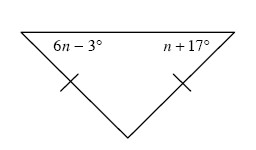2.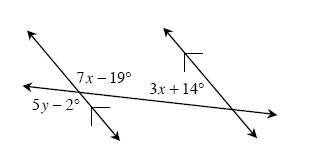3.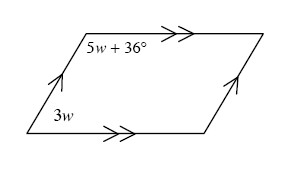4.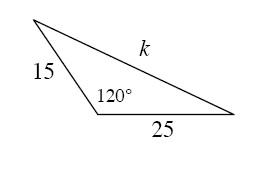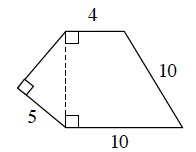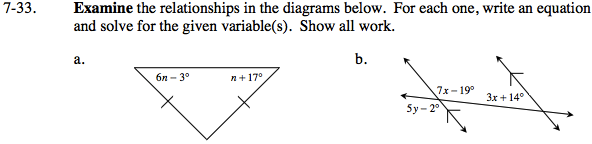Since the triangle is isosceles, the angles shown are equal.

n = 4°

First solve for x, then y. Refer to the Math Notes box in Lesson 2.1.4 if you need help remembering any specific angle pair relationships.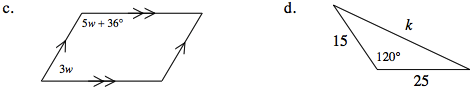Same side interior angles are supplementary.

w = 18°

Use the Law of Cosines to solve for k.